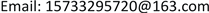﻿ 跨境电商第三方海外仓存储和物流成本研究 Research on the Third Party Overseas Storage Costs of Cross-Border Ecommerce

Management Science and Engineering
Vol. 07  No. 04 ( 2018 ), Article ID: 27722 , 6 pages
10.12677/MSE.2018.74028

Research on the Third Party Overseas Storage Costs of Cross-Border Ecommerce

Beibei Duan

School of Economics and Management, Beijing Jiaotong University, BeijingReceived: Nov. 3rd, 2018; accepted: Nov. 19th, 2018; published: Nov. 26th, 2018ABSTRACT

Taking the storage cost of cross-border e-commerce in the third-party overseas warehouse as the research object, the third-party overseas storage cost is divided into the first leg transportation costs, the comprehensive tariffs, the warehousing service costs, the out-of-stock loss costs and the local distribution costs. Based on the above four cost components, a mathematical model is established to calculate the economic order quantity under the condition of stochastic discretization and to find the appropriate order point and safety stock, which can provide a reference for the cross-border e-commerce to reduce the business cost.

Keywords:Cross-Border Electricity Supplier, Third-Party Overseas Warehouse, Storage Costs, Best Order Quantity1. 引言

2. 第三方海外仓储费用构成分析

3. 跨境电商第三方海外仓储成本模型

3.1. 头程运输成本C1

c11：一次订购费用。

c12：包括存储费用和出入库操作服务费用。根据单件货物体积和存储时间，按照一定费率收取的仓库存储和服务费用。

$\left\{\begin{array}{l}{c}_{12}=0+{m}_{o}+{n}_{o}×Q×{g}_{o}\text{\hspace{0.17em}}\text{\hspace{0.17em}}\text{\hspace{0.17em}}\text{\hspace{0.17em}}\text{\hspace{0.17em}}\text{\hspace{0.17em}}\text{\hspace{0.17em}}\text{\hspace{0.17em}}\text{\hspace{0.17em}}\text{\hspace{0.17em}}\text{\hspace{0.17em}}\text{\hspace{0.17em}}\text{\hspace{0.17em}}\text{\hspace{0.17em}}\text{\hspace{0.17em}}\text{\hspace{0.17em}}\text{\hspace{0.17em}}\text{\hspace{0.17em}}\text{\hspace{0.17em}}\text{\hspace{0.17em}}\text{\hspace{0.17em}}\text{\hspace{0.17em}}\text{\hspace{0.17em}}\text{\hspace{0.17em}}\text{\hspace{0.17em}}\text{\hspace{0.17em}}\text{\hspace{0.17em}}\text{\hspace{0.17em}}{t}^{1}\le {t}_{0}^{1};\text{\hspace{0.17em}}\text{\hspace{0.17em}}{t}_{0}^{1}\ge 0\\ {c}_{12}=Q×{K}_{i}^{1}×\left({t}^{1}-{t}_{0}^{1}\right)+{m}_{o}+{n}_{o}×Q×{g}_{o}\text{\hspace{0.17em}}\text{\hspace{0.17em}}\text{\hspace{0.17em}}\text{\hspace{0.17em}}\text{\hspace{0.17em}}\text{\hspace{0.17em}}\text{\hspace{0.17em}}\text{\hspace{0.17em}}{V}_{i-1}\le {v}_{o}\le {V}_{i}\end{array}$ (1)

${t}_{0}^{1}$ ：境内转运仓免仓租期(单位：天)；

t1：境内转运仓商品储存时间(单位：天)；

Q：订货批量；

Vi：境内转运仓单件货物体积计费区间上限；

Vi−1：境内转运仓单件货物体积计费区间下限；

${K}_{i}^{1}$ ：单件货物体积在 ${V}_{i-1}\le {V}_{0}\le {V}_{i}$ 区间之内的境内转运仓仓租费费率；

mo：境内转运仓入库作业费用常数；

no：境内转运仓每公斤货物入库作业费用；

go：单件货物重量。

c13：境内运输一般采用快递的方式，运输费用按照首重和续重的计费标准计费：

${c}_{13}={c}_{o}+{h}_{o}×Q×{g}_{o}$ (2)

co：从采购地到境内转运仓的首重费用；

ho：从采购地到境内转运仓的每公斤货物续重费用；

go：为单件货物重量。

c14：境内转运仓库到海外仓库的运输费用，根据货物的总体积的大小，采用不同的计算费率：

${c}_{14}=Q×{V}_{o}×{R}_{i},\text{\hspace{0.17em}}\text{\hspace{0.17em}}\text{\hspace{0.17em}}\text{\hspace{0.17em}}{V}_{1+i}^{1}\ge Q×{V}_{o}\ge {V}_{i}^{1}$ (3)

v0：单位产品体积；

${V}_{1+i}^{1}$ ：单件货物海运运费计费区间上限体积；

${V}_{i}^{1}$ ：单件货物海运运费计费区间下限体积；

Ri：一批货物体积数量在 ${V}_{1+i}^{1}\ge Q×{V}_{o}\ge {V}_{i}^{1}$ 区间内的每立方米货物海运运费。

${C}_{1}={c}_{1}×\frac{D}{Q}$ (4)

3.2. 综合关税C2

${C}_{2}={e}_{o}×\frac{D}{Q}$ (5)

eo：每次清关总费用。

3.3. 第三方海外仓储服务成本C3

$\left\{\begin{array}{l}{C}_{3}=0×\left(\frac{1}{2}×Q+S\right)\text{\hspace{0.17em}}\text{\hspace{0.17em}}\text{\hspace{0.17em}}\text{\hspace{0.17em}}\text{\hspace{0.17em}}\text{\hspace{0.17em}}\text{\hspace{0.17em}}\text{\hspace{0.17em}}\text{\hspace{0.17em}}\text{\hspace{0.17em}}\text{\hspace{0.17em}}\text{\hspace{0.17em}}\text{\hspace{0.17em}}\text{\hspace{0.17em}}\text{\hspace{0.17em}}\text{\hspace{0.17em}}\text{\hspace{0.17em}}\text{\hspace{0.17em}}\text{\hspace{0.17em}}\text{\hspace{0.17em}}\text{\hspace{0.17em}}\text{\hspace{0.17em}}\text{\hspace{0.17em}}\text{\hspace{0.17em}}\text{\hspace{0.17em}}{t}^{2}\le {t}_{0}^{2};\text{\hspace{0.17em}}\text{\hspace{0.17em}}{t}_{0}^{2}\ge 0\\ {C}_{3}={M}_{i}^{2}×\left({t}^{2}-{t}_{0}^{2}\right)×\left(\frac{1}{2}×Q+S\right)\text{\hspace{0.17em}}\text{\hspace{0.17em}}\text{\hspace{0.17em}}\text{\hspace{0.17em}}\text{\hspace{0.17em}}\text{\hspace{0.17em}}\text{\hspace{0.17em}}\text{\hspace{0.17em}}\text{\hspace{0.17em}}{t}^{2}>{t}_{0}^{2};\text{\hspace{0.17em}}\text{\hspace{0.17em}}{V}_{i-1}^{2}\le {v}_{o}\le {V}_{i}^{2}\end{array}$ (6)

${M}_{i}^{2}$ ：表示海外仓仓租费率；

${t}_{0}^{2}$ ：表示海外仓面仓租期(单位：天)；

${V}_{i}^{2}$ ：表示单件货物海外仓存储费计费区间上限体积；

${V}_{i-1}^{2}$ ：表示单件货物海外仓存储费计费区间下限体积。

3.4. 缺货损失C4

$Y={D}_{Y}+S=\mu ×\omega +S$ (7)

${F}_{x}\left(d\right)=\frac{{\text{e}}^{-x}{\left(x\right)}^{d}}{d!}$ (8)

${Q}_{Y}={\sum }_{d=Y+1}^{\infty }{\sum }_{x=\mu -\epsilon }^{\mu +\epsilon }P\left(x\right)×{F}_{x}\left(d\right)×d$ (9)

${C}_{4}=\frac{D}{Q}×f×{Q}_{Y}$ (10)

3.5. 总成本C

$C={C}_{1}+{C}_{2}+{C}_{3}+{C}_{4}$ (11)

4. 实证分析

4.1. 头程运输成本

${C}_{1}=148×\frac{D}{Q}+4.4×D$

4.2. 综合关税

${C}_{2}={e}_{o}×\frac{D}{Q}=72×\frac{D}{Q}$

4.3. 海外仓存储服务费用

${C}_{3}=16.5×\left(\frac{1}{2}×Q+S\right)$

4.4. 缺货损失

$P\left(x\right)=\frac{1}{\sqrt{2\text{π}\sigma }}{\text{e}}^{-{\left(x-20\right)}^{2}/2}$

${Q}_{Y}={\sum }_{d=Y+1}^{Y+15}{\sum }_{x=18}^{22}P\left(x\right)×{F}_{x}\left(d\right)×d$

${C}_{4}=\frac{D}{Q}×250×{Q}_{Y}$

$C={C}_{1}+{C}_{2}+{C}_{3}+{C}_{4}=\left(148+72+250×{Q}_{Y}\right)×\frac{D}{Q}+8.25×Q+4.4×D+16.5×S$Table 1. Calculation table of order point, safety stock, best order quantity, and cost

5. 结论

Research on the Third Party Overseas Storage Costs of Cross-Border Ecommerce[J]. 管理科学与工程, 2018, 07(04): 244-249. https://doi.org/10.12677/MSE.2018.74028

1. 1. 孟亮, 孟京. 我国跨境电商企业海外仓模式选择分析——基于消费品出口贸易视角[J]. 中国流通经济, 2017, 31(6): 37-44.

2. 2. 宋海英, 许琳. 海外仓——电商时代物流业发展的必然趋势[J]. 全国商情(经济理论研究), 2015(3): 24-25.

3. 3. 钱松迪. 运筹学[M]. 北京: 清华大学出版社, 2012.

4. 4. 秦新生. 跨境电商企业海外仓储成本合理化方案[J]. 对外经贸, 2017(8): 38-40.

5. 5. 高本河. 供应链管理[M]. 深圳: 海天出版社, 2004.

6. 6. 李双双. 考虑提前期压缩的动态供应链鲁棒控制研究[D]: [硕士学位论文]. 哈尔滨: 哈尔滨商业大学, 2017.

7. 7. 豆丁网. 递四方速递海外仓报价表[EB/OL]. http://www.docin.com/p-945331029.html, 2018-01-29.